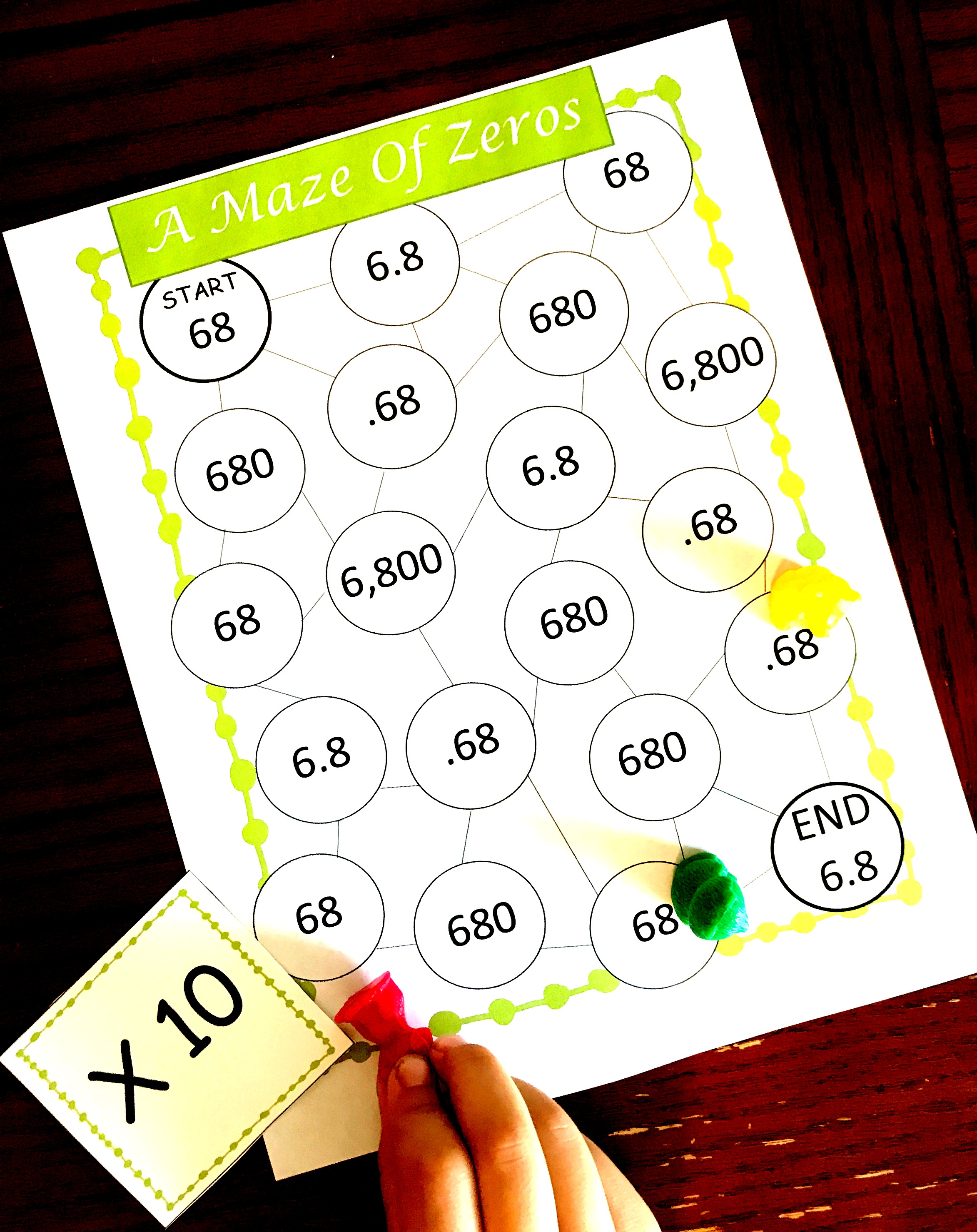# Multiplying by Powers of 10 | Free Game | Decimals

## Multiplying by Powers of 10

Here is a free game to practice multiplying by powers of 10. Students will practice using .10 or 10. This free game will help your students practice this concept or help you teach 5.NBT.A.1.

This week I’m doing a 3-part series on place value with decimals or multiplying by 10 and 1/10.

In the first post, we talked about what happens when you move one place to the right, you are multiplying by 1/10, and if you move one place value to the left you are multiplying by 10.

You can see the posts below:

Multiplying Decimals

## Multiplying with Decimals Games

Children are laughing and having animated discussions. You can see their happy faces, hear excited voices, and the loud groans from drawing the wrong card.

No, you aren’t out on a playground, you are in a math classroom where the students are playing math games.

## Multiplying by 10

Of course, we talked about getting our base ten blocks and letting the children explore. What may have worked even better for you was to get out grid paper.

This allows your students to actually cut apart a long or flat section so they can multiply by 10 or .10.

The next post in the series will provide your students with extra pencil and paper practice with an assessment by teaching students place value with decimals.

Before that, let’s practice in a way that will get some smiles on the faces of your kiddos.

## Multiplying with Decimals Game

1. First, glue the game board onto a file folder.
2. Next, cut out the cards that have the operations on them.
3. Finally, gather up a few game board pieces.

## How to play a Maze of Zeros

The concept is simple. The children start at a number like 25 and then draw a card that will have them multiply by 10 or .10. If there is a line to the correct answer they may move their game piece to the next circle.

If not, they must remain on their current number. The goal is to be the first person to reach the end of the maze.

Enjoy the sounds of your students having fun while playing the multiplying by 10 game.

## Multiplying by 10 Worksheet

Below you will find the multiplying by 10 worksheets. It will help teach multiplying by 10 through a fun and entertaining game. If you need more decimal practice use this Decimal Multiplication Using A Lattice Grid Practice Worksheet.

Multiplying-By-1_10-and-10